Reach Us+44-1522-440391
Detection of Thyroid Cancer Clusters in Algeria | OMICS International
Journal of Biometrics & Biostatistics

Like us on:

All submissions of the EM system will be redirected to Online Manuscript Submission System. Authors are requested to submit articles directly to Online Manuscript Submission System of respective journal.

# Detection of Thyroid Cancer Clusters in Algeria

Moussi O1*, Boudrissa N1, Bouakline S2, Semrouni M3 and Hasbellaoui F3

1National School of Statistics and Applied Economics D' Alger, 11, chemin doudou Mokhtar, Ben Aknoun, Algeria

2University of Bejaia - Campus Targa Ouzemour, Bejaia, Algeria

3Centre Pierre and Marie Curie, Social and Medical Centres, Algeria

*Corresponding Author:
Oumelkheir Moussi
National School of Statistics and Applied Economics D' Alger, 11
chemin doudou Mokhtar, Ben Aknoun, Algeria
Tel: +213 21 91 21 33
E-mail: [email protected]

Received date: February 23, 2015; Accepted date: May 22, 2015; Published date: May 29, 2015

Citation: Moussi O, Boudrissa N, Bouakline S, Semrouni M, Hasbellaoui F (2015) Detection of Thyroid Cancer Clusters in Algeria. J Biomet Biostat 6:225. doi: 10.4172/2155-6180.1000225

Copyright: © 2015 Moussi O, et al. This is an open-access article distributed under the terms of the Creative Commons Attribution License, which permits unrestricted use, distribution, and reproduction in any medium, provided the original author and source are are credited.

Visit for more related articles at Journal of Biometrics & Biostatistics

#### Abstract

In the mid-seventies, the Pierre and Marie Curie Center of Algiers (CPMC), was the only structure at the national level to take care of patients with thyroid cancer, it recorded from 15 to 20 cases per year. Today, and although Algeria has ten structures for the management of these patients, CPMC has recorded more than 100 new cases of thyroid cancer per year over the period 2007/2011 This disease is the third most common cancer for women. These observations lead us to ask several questions: • Are there wilayas having an excessive number of cancer’s cases? • Is cases’ concentration abnormally? • Is the spatial distribution of these cases random? Answering these questions is by describing the spatial heterogeneity, that is to say, identifying potential spatial clusters. A cluster is spatial organization defined as an aggregation, a combination of the nearest cases, the proximity being defined in the sense of geographical distance. Various statistical methods have been used to study the spatial heterogeneity. The global methods, for the detection of clusters, the study of the spatial correlation, and the detection of cases tending to "clustering", and the local methods, for the identification of clusters of cases inconsistent under the null hypothesis of no clusters, and the evaluation of their significance level. To study the spatial distribution of the disease, several tests were applied, such as the test of Pearson, the index of Moran, the Tango test, and Pothoff-Whittinghill test to check the hypothesis of constant risk of the incidence of thyroid cancer and study the tendency to "clustering", next the "Two-step clustering", was used for the localization of the clusters, and the scanning Kulldorf test to identify potential clusters, confirm and assess their significance.

#### Keywords

Cluster; Cancer; Thyroid; Test; Moran; Tango; Kulldorf

#### Introduction

In the mid-seventies, the Pierre and Marie Curie Center of Algiers (CPMC) was the only structure at the national level to support patients with thyroid cancer, it recorded from 15 to 20 cases per year. Today, even if Algeria has 10 structures for the management of these patients, which are from one of the forty eight wilayas (states), the CPMC recorded more than 250 new cases of thyroid cancer per year between 2007 and 2011 and it is the third cancer for woman according to the tumors register of the Algiers. These observations lead us to ask the following questions:

• Which wilayas have an excessive number of thyroid cancer cases? And therefore are most excessive cases abnormally concentrated in these regions?

• Is the spatial distribution of these cases random?

The answering of these questions is by describing the spatial heterogeneity; that is to say, identifying possible spatial clusters or aggregates.

A cluster is a spatial organization defined as an aggregate, a grouping of the cases which are close to each other; the proximity being defined in the sense of geographical distance [1,2]. Various statistical methods  have been used to study the spatial heterogeneity.

Global cluster detection methods , for studying the spatial correlation and detecting the trend of the events to "clustering", and the local cluster detection methods  for identifying the incompatible combinations of cases with the null hypothesis of no clustering, and evaluating their significance level.

In the literature, it does not exist a direct method for understanding the spatial distribution of a disease, for this reason we favored the application of several tests, based on assumptions and different methodologies (as suggested by Huang et al. ; the goal being the convergence of the results. Pearson, Moran, Tango and Pothoff- Whittinghill tests were used to verify the constant risk assumption, and seek for the tendency to "clustering", while the "Two-step cluster" method and Kulldorff scanning method were utilized to identify potential clusters, confirm and assess their significance.

#### Review of Literature

The statistical tools used in epidemiology knew a considerable development since the late 1980, and particularly through the expansion of computers and simulation methods. The development of these approaches was done by the consideration of possible spatial autocorrelation which was not taken into account in the classical models as the Poisson model. Spatial autocorrelation is defined by the similarity of incidence values for neighboring areas: the risk of disease in a geographic area is not independent of the neighboring areas. Significant spatial autocorrelation can be explained by the tendency of data to aggregation (clustering).

Several studies have been developed [3,5,6] to test the tendency for aggregation of cases of a pathology. Their goal was the better understanding of the geographical distribution of a disease, and the study of the spatial heterogeneity.

A cluster or an aggregate can be defined as a typical concentration of a group of people, in a geographical area or/and a time period.

The proposed tests aims to know if the events aggregated in a space can be classified according to their purpose. We distinguish between three kinds of tests: global tests which assess the overall tendency to aggregation (clustering) of the incidence of a disease in a studied area, the tests for local detection of clusters, and finally the focused tests which are used when a prior information exists, and allows the specification of the geographic coordinates which are the focus of the search in order to seek for a case concentration.

If the methods of "global clustering" study the spatial correlation and the detection of the tendency of cases to aggregation, the local methods of detecting clusters identify the cases of incompatible combinations with the null hypothesis "lack of clustering", and assess their level of significance.

Cluster analysis can be classified according to the type of data under study, these to be aggregated (the number of cases, and the population per municipality in the studied geographical area, such as the case in this project) either specific or individual (the spatial coordinates of cases, and population at risk or witnesses).

To perform the global tests, it is necessary to describe the proximity between spatial units, the latter being given by a matrix called the proximity (denoted by W) which is a squared matrix summarizing the relationship between each pair of the spatial units, and the studied area. This proximity may be the distance between two spatial units, or whether the couple share borders or not [3,6]. This allows us to assign a weight to each pair of the spatial units.

Local Detection of Clusters and Spatial Scanning Methods

The purpose of the spatial scanning methods is monitoring the geographical territory in order to detect areas where a high incidence of cases of a disease is observed, without prior assumptions. They seek to put together the various neighbouring spatial units of the studied area within potential clusters. They apply windows (circles or ellipses) throughout the region, and count the cases and individuals at risk inside and outside of each window. There are different spatial scanning methods including the method of Besag and Newell  and the spatial scan statistic of Kulldorff [8,9]. These methods are distinguished by the construction of the window they use.

The spatial scan statistic of Kulldorff [8-10] is the most used one. It relies on a window, called scanning, moving geographically. This window is placed at different coordinates (X, Y) (the centroid of the studied units) with a radius that varies from 0 to a predefined limit, based on the size of the population (usually the window should not cover more than 50% the studied population).

The general principle of the method is defined as follows: for each scanned location, we assume that the probability p that a point to be a case within the window is equals to the probability 'q' that a point in outside the window to be a case, this means that the points is randomly distributed. Alternatively if for each spatial position and window size, the risk inside the window is higher than it outside; then all the cases included in this window compose a potential cluster.

The likelihood functions are written according to the probability distribution associated with the number of cases. Two distributions can be used: the Poisson distribution (when the number of cases is too small compared with the population size), and the binomial distribution (when individual data are available about the cases and the witnesses).

The statistical test is based on the likelihood ratio. Under the assumption of a Poisson distribution of the number of cases, the most likely cluster corresponds to the circle for which the likelihood ratio given in the following equation is maximum: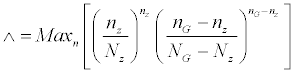(1)

The Kulldorff method allows the organization of clusters according to their likelihood ratio, and identifies the secondary clusters. The value of the degree of significance of the clusters is obtained by iterating the likelihood ratio using Monte Carlo simulation.

The SaT Scan software  can be used to implement the spatial scan and space-time scan statistics. This software was developed by Kulldorff, it detects spatial clusters and spatiotemporal clusters, it verifies if they are statistically significant; in order to test whether the disease is randomly distributed in space or/and time or not, and it performs regular monitoring of a disease in a geographic area.

The number of cases, the population frequencies, and the geographic coordinates of each spatial unit (in our project the capital of the states) of the studied area must be defined. The covariates like (gender, age groups, population density, socio-economic score ...) can be also used. The maximum size of the cluster must be defined in function of the population size.

The advantages of this method are: the incorporation of the covariates in the analysis, and a total value of significance is provided for the test, the location even the approximate location of the cluster that causes the rejection of the null hypothesis is given.

The spatial distribution of the studied area (and the temporal distribution of the studied time period) has an effect on the detected clusters. The spatial scan statistic tends to detect clusters larger than that of the true clusters encompassing neighbouring areas where there is no high risk.

Other clusters detection methods have been developed especially to detect clusters of arbitrary shape. However, the spatial scan method of Kulldorff is the most used tool to identify potential clusters.

Global tests for the clusters’ detection

These methods are concerned with the existence of an overall uneven spatial distribution of disease. Their objective is to study the over-dispersion, the spatial correlation and detect the trend of cases to "clustering" but they don’t give the location of the clusters.

There are many approaches for global cluster detection. We present here the chi-square test of Pearson, Potthoff and Whittinghill test, and Moran and Tango tests that are widely used in spatial correlation studies.

Chi-square test of Pearson: This method is used to test the existence of a global spatial heterogeneity in terms of over-dispersion. Instead of using a spatial autocorrelation coefficient, some authors propose to fit a statistic estimating the discrepancy between the observed and the theoretical values from a probabilistic model. The chi-square (χ2) statistic of Pearson given by: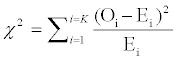(2)

Where:

K= the number of spatial units, Oi=the number of observed cases in the spatial unit.

Ei=the number of expected cases in the spatial unite i, under H0

Under H0 (or non-attendance of clusters), the expected number of cases (Ei) is randomly distributed according to a Poisson model and the χ2 statistic follows a chi-square distribution with (K-1) degrees of freedom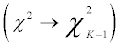The rejection of the constant risk assumption or the Poisson random model suggests the existence of clusters. The χ2 statistic of the test provides an acceptable overall cluster detection but it is not able to locate the spatial character using the differences according to the theoretical model, that is to say, if several spatial units have significant deviation from the theoretical model, the statistic remains unchanged even if these spatial units are contiguous (suggesting a cluster) or not [2,12].

Tango statistic: Tango , proposed a statistic for evaluating a global clustering. The method of Tango tests if the disease cases are grouped in clusters within the studied area. He generalized spatially the chi-square test of Pearson by weighting the differences with the proximity. The Tango statistic (denoted by T) is defined as: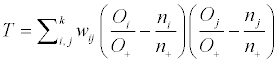(3)

Where: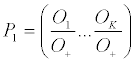is the vector of the observed proportions,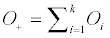is the total number of observed cases, and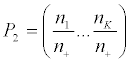is the vector of the expected proportions with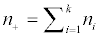the total number of population at risk.

Under the null hypothesis of constant risk, the expected proportions are supposed to come from a multinomial distribution, and the variable T is asymptotically normal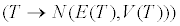with a fairly low speed of convergence [3,14].

With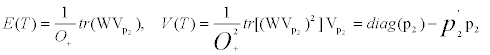The standard form (score) of Tango Statistic is given by: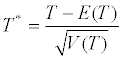(4)

Tango proposed an approximation using the chi-square law of the statistic T*. Under the hypothesis of constant risk: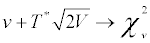(5)

And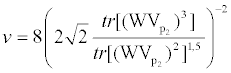(6)

Global moran statistic: The second method measuring the existence of the global spatial heterogeneity in terms of spatial autocorrelation. The Moran Statistic is the most used index of spatial autocorrelation. This statistic summarizes the degree of resemblance of the neighbouring geographic units by a weighted average of the similarity between observations [12,15].

The test is based on the well-known Moran statistic or index, denoted I is defined by: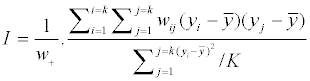(7)

Where:

K=the number of spatial units.

wij=the elements of proximity matrix for the spatial units i and j.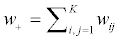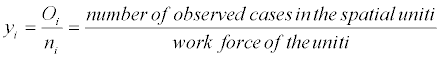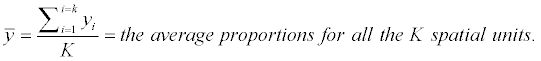The Moran statistic I is a random variable which follows (under the hypothesis of constant risk), an asymptotically normal distribution whatever the spatial unit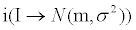with: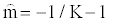(8)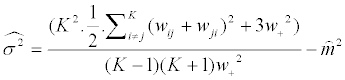(9)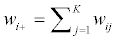(10)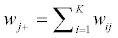(11)

The Moran index measures the similarity between the neighbouring spatial units, its interpretation is similar to that of a correlation coefficient .

• I<0 ⇔ negative spatial autocorrelation, so neighbouring spatial units are different.

• I ≃ 0 ⇔ there is no correlation between neighbouring spatial units, and the spatial model is perfectly random.

• I>0 ⇔ The neighbouring spatial units are similar (the existence of a pattern as a cluster of spatial units).

Moran statistic doesn’t take into account the heterogeneity of the population frequencies, a significant spatial correlation could be explained by the proximity of densely populated areas and not by a cluster with high rates. Alternative versions of the Moran statistic have been proposed to take into account the frequencies of heterogeneous population.

Potthoff-Whittinghill test: The test for heterogeneity (over dispersion) of Potthoff-Whittinghill is more powerful in the case of low heterogeneity, and it is frequently used in epidemiology. Under the null hypothesis of a random distribution of the cases of a disease, the incidence rates are the same throughout the area under study, and the changes in the observed cases are only related to the changes in the law of probability. The number of the observed cases is assumed to follow a Poisson distribution with mean and variance equals to the number of expected cases.

Under the alternative hypothesis of the existence of over dispersion of cases, the number of cases is higher in some areas than what is expected under the assumption of a Poisson distribution .

This test checks whether the dispersion of incidence rate is considered too high to be compatible with the random fluctuations of Poisson.

The test Potthoff and Whittinghill is based on the statistic: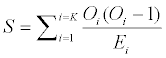(12)

Under the null hypothesis of constant risk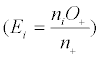, the S statistic converges in law to a normal distribution with mean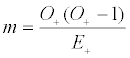and variance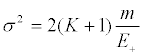E+ is the total number of cases expected under the constant risk hypothesis.

The DCluster package (from R) can be used to perform the test of Potthoff-Whittinghill.

Identification of clusters

The purpose of an analysis of clusters is to create homogeneous groups of individuals according to a number of variables. Specifically, we want to combine interpretable subject groups, so that individuals of the same group are similar and the groups are different. Several methods have been developed for the treatment of continuous data and others for the qualitative data, but these methods are unreliable for the two types of data at the same time (the most frequent case in practice) [17,18].

The method of clusters in two steps (Two-step clustering) introduced by Chiu et al.  is to identify clusters for mixed data. So the data will be organized into groups (clusters), the members of each cluster are very similar to each other and very dissimilar with the members of other clusters; this is based on one or more discriminate variables. The name of the method means that the algorithm is applied in two steps:

Step 1: the individuals are assigned to pre-clusters.

Step 2: Pre-clusters are clustered a second time using the hierarchical algorithm .

This method is implemented in the SPSS package and it assumes that all the variables are independent, the continuous variables are normally distributed, and the categorical ones are multinomial.

In summary, the methods in 2-2 and 2-3 serve firstly to test whether the distribution of cases is random in the study area, and also to detect areas where there is an abnormal concentration of patients. Huang et al.  compare these different tests to determine the most appropriate method or (and) the most powerful one to understand the spatial distribution of a disease, and to provide a guide for the use of these statistical methods when they are applied to cancer data.

Among the tests for "clustering" of overall consideration, the Tango test seems the most potent. Among the local cluster detection methods, the Kulldorff statistic with elliptical windows seems to be the most powerful .The other methods, can’t be considered as "screening" methods and must be completed by more targeted studies to confirm or refute the hypotheses they generate. In this context, the use of multiple tests, based on assumptions and methods of different estimates with convergence of results, would be a solution.

This is the methodology adopted in the application part, in order to seek the convergence of the results by the use of multiple tests. We started by looking for a tendency to clustering (Pearson tests, Tango, Pothoff and Whittinghill), followed by the search for potential clusters (two-step clustering) and finally identify and locate the clusters which are statistically significant (approach Kulldorff).

#### Application

Data description

The study is based on 527 hospitalized patient originated from one of the forty-eight wilayas of Algeria (from which 98% are women), and subjected to chirurgical operation on the thyroid cancer, in the endocrinology service at the Pierre and Marie Curie Center (CPMC) of the Mustapha Bacha hospital located in Algiers for the time interval 2007-2011. Data includes the number of cases per wilaya (Geographical origin), the population at risk, and therefore the incidence [20,21].

Methods and softwares

The Excel was used to calculate the chi-square statistic of Pearson, Moran index, Tango statistic, and at last Pothoff-Wittinghill statistic. The proximity matrix used in the study is the adjacency matrix because the only information available is the geographical origin. It is defined by: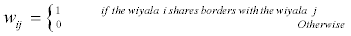(13)

It was assumed that the wilaya i has no borders with itself, and this means thatwii=0. This procedure facilitated the calculations because the resulting matrix is symmetric.

The Easy Fit software was used to test the goodness of fit of the Poisson distribution to number of patients, and the SPSS package to identify the potential clusters of thyroid cancer using the two-step cluster method as described previously.

The SatScan software was used to confirm the existence of clusters and their significance. This software allows us to apply the scan method of Kulldorff , which consists in grouping together the different neighboring units (wilayas) within a significant cluster [22-24].

#### Results

From Table 1 we observe that:

Score Calculated Value Critical Value Significance level
Pearson 150.98 64.001 5%
Moran 5.73 1.65 5%
Tango 307.902 21.026 5%
Pothoff-Whittinghill 39.825 1.65 5%
Goodness of fit test 63 × 10^12 7.83 5%

Table 1: The results of the global tests.

The score of the Pearson chi-square (χ2=150.598) is strictly greater than its critical value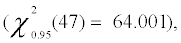so the Poisson distribution doesn’t fit the observed number of patients, then we reject the constant risk assumption, and this was confirmed by the goodness of fit test.

Moran’s index (I=0.4913) is positive and greater than its theoretical mean value (E(I)=-0.0212), it induces the score 5.73 which is greater than the critical value 1.65; then we accept the hypothesis of spatial heterogeneity at 5%, significance level.

Tango statistic is equal to T=0.043, and the approximation by the law of chi-square is equal to 307.902 which is greater than the 95% quintile of a chi-square at 12 degrees of freedom, then we reject the homogeneity assumption.

Pothoff and Wittinghill statistic is equal to 39.825, which is greater than the critical value of the test this implies a heterogeneous distribution of the observed cases.

The results of the global tests indicate that there is a tendency to aggregate the cases of thyroid cancer, and suggests the possibility of the existence of potential clusters. Then we will try to locate them using the two-step cluster analysis and the spatial scan statistic.

The two-step clustering method reveals three homogenous groups (thus three potential clusters) with respect to the variable incidence, the results are given in Table 2:

Potential clusters The wilayas within the cluster
1 Adrar,Laghouat,Batna,Tebessa,Tlemcen,Tiaret,Djelfa,Sétif,Saida,Sidibelabbes,Annaba,GhardaiaMascara, Ouargla, ElTarf, Tindouf, Tissemsilt, Khenchela, SoukAhras,AinDefla, Ghardaia
3 Chlef,  Béjaia,  Blida,  Bouira,  Tizi-Ouzou, Alger,  Médéa,  M’Sila,  Illizi, Bordj-Bou- Arrérij, Boumerdes, Tipaza

Table 2: Potential clusters by the two-step cluster method.

The Kulldorff approach was applied too via the SatScan package and it highlights one significant spatial cluster with a very small p-value equals to p<10-17 (which is the probability that the cluster is not significant). This cluster includes the wilayas Alger, Boumerdes, Blida, Bouira, Médéa, Tipaza, Tizi-Ouzou, Ain-Defla, Bordj-Bou- Arrérij ,M’Sila, Chlef, Bougie. It contains 389 cases over a radius of 179.9 kilometers and the relative risk is equal to 5. (See the distribution on map for this cluster in the appendix).

When the time factor was introduced, it was found that there is only one significant temporal cluster between 2007/2011 involving 441 cases, with a relative risk of 8.82 and a p-value equal to 0.001. (See the appendix)

Including the two factors at the same time (space and time) yielded one significant temporal-space cluster between 2007 and 2011 involving the wilayas Alger, Boumerdes, Blida, Bouira, Médéa, Tipaza, Tizi-Ouzou, Ain-Defla, Bordj-Bou-Arrérij, M’Sila, Chlef, Tissemsilt, Bougie, Sétif, Tiaret, Jijel, Djelfa, it contains 371 cases with a relative risk of 3.78, and a very small p-value (p<10-17). (See this cluster on map in the appendix).

#### Discussion of Results

All the applied statistical methods reject the constant risk assumption, and confirm the existence of a non-random pattern. Pearson test, Moran index, Tango and Pothoff-Whittinghil tests show a significant tendency to clustering.

The "two-step clustering" approach highlights three potential clusters. The Cluster three was confirmed as significant spatial one without the wilaya of Illizi by the spatial scan statistic. This could be explained by the fact that it takes into account the geographical proximity. Moreover, this same cluster (without Illizi) is confirmed as spatio-temporal cluster.

Then the different used methods converge toward the same result, that there's an abnormal concentration of cases of thyroid cancer in the region encompassing the wilaya of Algiers, Chlef, Bejaia, Blida, Bouira, Tizi-Ouzou, Medea, M'Sila, BordjBouArrerij, Boumerdes, Tipaza, AinDefla, Tissemsilt. This area is a spatial and spatio-temporal cluster with a relative risk of 5.01; that is to say, residing in these wilayas increases the risk of having a thyroid cancer by five, comparing with the other ones.

#### Conclusion

The applied statistical methods converge toward the same conclusion: they reject the assumption of a constant risk, then the distribution of cases is not random, and there exists an unusual concentration of cases of thyroid cancer in some wilayas of Algeria, especially in Algiers, Chlef, Bejaia, Blida, Bouira, Tizi-Ouzou, Medea, M'Sila, Bordj BouArrerij, Boumerdes, Tipaza, Ain Defla, Tiaret, Tissemsilt. This Cluster is simultaneously a spatial and spatio-temporal over the period 2007/2011.

Although the CPMC remains the biggest recruitment center of the thyroid cancer, over the same period other hospitals also took care of patients with the same pathology. So the incidence rate could only increase and other potential clusters could emerge if the study is widespread nationally.

It appears from this study that some wilayas such as (Chlef, Bejaia, Tizi-Ouzou, Medea, and Bouira) belonging to the significant spatial cluster are already known as endemic goiter, and this is due to the considerable lack in the consumption of the iodine for this population. This deficiency was supported and prophylactic measures were implemented (such as the obligatory sale of iodized salt made compulsory by the decree 90-04 of 20/01/1990). But in 1992 and according to the professor Moulay Ben Miloud (2008, The congress of Algerian Endocrinological Society) 90% of Algerian households used iodized salt through an awareness program, but later this percentage have declined reaching between 50 and 60% only. Indeed the iodization of the salt increases its cost, and this lead some retailers to commercialize a non-iodized salt (in 2012, 128 pounds of non-iodized salt were seized on sale in several food stores).

The lack of iodine, leads to a nodular goiter and the thyroid cancer develops in this kind of goiter. Moreover, if the amount of iodine exceeds the standards, the thyroid function will become misaligned, and this causes the abnormalities associated with papillary thyroid cancer in about 30% of cases (The most prevalent kind of the thyroid cancer).

This analysis is a preliminary work and must be followed by the investigation of the environmental and the socio-economic risk factors associated with this disease, using epidemiological surveys to get more information, especially in the wilayas of the significant cluster.

#### References

Select your language of interest to view the total content in your interested language

### Article Usage

• Total views: 12066
• [From(publication date):
April-2015 - Jul 20, 2019]
• Breakdown by view type
• HTML page views : 8278Can't read the image? click here to refresh